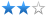﻿ PL/SQL Record and Table Type Definition - Alibaba Cloud Developer Forums: Cloud Discussion Forums
﻿
Engineer• UID623
• Fans4
• Follows1
• Posts72

# [PostgreSQL Development]PL/SQL Record and Table Type Definition

Created#
More Posted time:Aug 19, 2016 10:59 AM
Oracle PL/SQL is a powerful SQL programming language. Many Oracle users use it to process some service logic that requires a low delay and high data consistency or reliability.
PostgreSQL also provides an advanced built-in SQL programming language, namely PLPGSQL. PostgreSQL PLPGSQL and Oracle PL/SQL employ similar syntax, with a few differences.
(P.S.: In addition to PLPGSQL, PostgreSQL uses popular languages including C, Java, Python, and Perl as function programming languages.)
This article provides some solutions for Oracle users who encounter function syntax compatibility issues when using PostgreSQL.
The solutions cover the syntax for the type xx is table of xxxx index by binary_integer and type xx is record functions.
Oracle PL/SQL Example

CREATE OR REPLACE FUNCTION f_xml(p_xml CLOB) RETURN INT
AS
...
type rec_tk is record
(
tkno VARCHAR2(100) ,
cg_zdj number(12,0) := 0 ,
cg_jsf number(12,0) := 0
);

type tklist is table of rec_tk index by binary_integer;

type rec_cjr is record
(
cjrid varchar2(30) ,
tk tklist
);

type cjr is table of rec_cjr index by binary_integer;
p_cjrs cjr;

FOR j IN 0..v_nllen-1 LOOP
BEGIN
...
p_cjrs(j).cjrid := v_nodevalue;
...
p_cjrs(j).tk(v_tkcount).tkno := v_nodevalue;
p_cjrs(j).tk(v_tkcount).cg_zdj := nvl(v_nodevalue,0);
p_cjrs(j).tk(v_tkcount).cg_jsf := nvl(v_nodevalue,0);
...
v_tkcount:=v_tkcount+1;

END LOOP;

This example involves the type and type table definitions supported by Oracle PL/SQL. PostgreSQL uses other type and type table definitions.
Example of Non-Compatibility Between PostgreSQL and PL/SQL
For PostgreSQL, types must be defined in a database instead of a function.
The functions involved in the preceding example must be adjusted as follows when they are used in PLPGSQL:
1.

type rec_tk is record
(
tkno VARCHAR2(100) ,
cg_zdj number(12,0) := 0 ,
cg_jsf number(12,0) := 0
);

type tklist is table of rec_tk index by binary_integer;\

It must be changed as follows:
SQL statement for type creation without function involvement

create type rec_tk as
(
tkno VARCHAR(100) ,
cg_zdj numeric(12,0) ,
cg_jsf numeric(12,0)
);

2.

type rec_cjr is record
(
cjrid varchar2(30) ,
tk tklist
);

type cjr is table of rec_cjr index by binary_integer;
p_cjrs cjr;

It must be changed as follows:
SQL statement for type creation without function involvement

create type rec_cjr as
(
cjrid varchar(30) ,
tk rec_tk[]
);

A function uses a table by using an array. The subscript for array reference begins from 1.

p_cjrs rec_cjr[];

3.

p_cjrs(j).cjrid := v_nodevalue;
...
p_cjrs(j).tk(v_tkcount).tkno := v_nodevalue;
p_cjrs(j).tk(v_tkcount).cg_zdj := nvl(v_nodevalue,0);
p_cjrs(j).tk(v_tkcount).cg_jsf := nvl(v_nodevalue,0);

Currently, PLPGSQL cannot directly change composite elements corresponding to a composite array.
It must be changed as follows:

declare
v_p_cjrs rec_cjr;
v_tk rec_tk;
...
v_p_cjrs.cjrid := v_nodevalue;
p_cjrs[j] := v_p_cjrs.cjrid;
...
v_tk.tkno := v_nodevalue;
v_tk.cg_zdj := nvl(v_nodevalue,0);
v_tk.cg_jsf := nvl(v_nodevalue,0);
v_p_cjrs.tk[v_tkcount] := v_tk;
p_cjrs[j] := v_p_cjrs;

Alternatively, change it with reference to the following example:

do language plpgsql \$\$
declare
vtk rec_tk;
vtk_a rec_tk[];
vcjr rec_cjr;
vcjr_a rec_cjr[];
begin
vtk := row('a', 1,2);
-- or vtk.tkno := 'a'; vtk.cg_zdj := 1; vtk.cg_jsf := 2;
vtk_a := vtk;

vcjr := row('test', vtk_a);
-- or vcjr := row('test', array[row('a',1,2)]);
-- or vcjr.cjrid := 'test'; vcjr.tk := vtk_a;
-- or vcjr_a := row('test', array[row('a',1,2)]);
vcjr_a := vcjr;
raise notice 'vtk %, vtk_a % vcjr % vcjr_a % ', vtk, vtk_a, vcjr, vcjr_a;
end;
\$\$;

NOTICE:  00000: vtk (a,1,2), vtk_a {"(a,1,2)"} vcjr (test,"{""(a,1,2)""}") vcjr_a {"(test,\"{\"\"(a,1,2)\"\"}\")"}
LOCATION:  exec_stmt_raise, pl_exec.c:3216
DO

For details of the nvl function, refer to Oracle's orafce package for compatibility with PostgreSQL.

4. Loop

[ <<label>> ]
FOREACH target [ SLICE number ] IN ARRAY expression LOOP
statements
END LOOP [ label ];

Row and column conversion

unnest(ARRAY[1,2])
1
2;

Summary
1.        Composite types is used to replace type definitions in PL/SQL.
2.        Arrays are used to replace table definitions in PL/SQL.
3.        Composite elements of composite arrays cannot be directly modified. You need to modify scalar values and then modify the elements by assigning the values.
RDS PG Kernel Optimization Suggestion
1.        Add the CREATE TYPE [ IF NOT EXISTS ] syntax, so that users do not need to write the syntax outside a function but can directly use it within a function.
2.        type is a local variable for PL/SQL while it is a global variable for PostgreSQL. If multiple PL/SQL functions use the same type name and different structures, multiple types must be created when the code is ported to PLPGSQL.
3.        Currently, PLPGSQL does not allow setting element values directory for composite arrays. Therefore, the syntax of PLPGSQL must be improved.
[Adolph edited the post at Aug 19, 2016 12:51 PM]You need to login to reply the post, Please log in or register
﻿